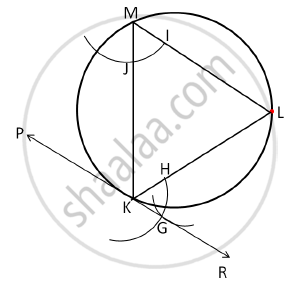# Draw a circle of radius 3.5 cm. Take any point K on it. Draw a tangent to the circle at K without using centre of the circle - Geometry

Draw a circle of radius 3.5 cm. Take any point K on it. Draw a tangent to the circle at K without using centre of the circle.

#### Solution

Steps of construction:
1. Draw a circle of radius 3.5 cm. Take any point K on it.
2. Draw a chord KL through K. Take any point M on the major arc KL.
3. Join KM and ML.
4. Draw an arc of the same radius taking M and K as the centres. Taking H as the centre and radius equal to IJ, draw an arc intersecting the previous arc at G.
∴ ∠KML = ∠LKR
5. Join KG and extend it on both the sides to draw PR which is the required tangent to the circle at K.Concept: Construction of Tangent Without Using Centre
Is there an error in this question or solution?